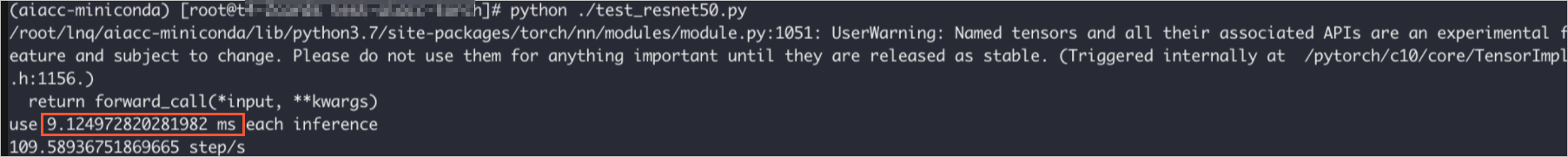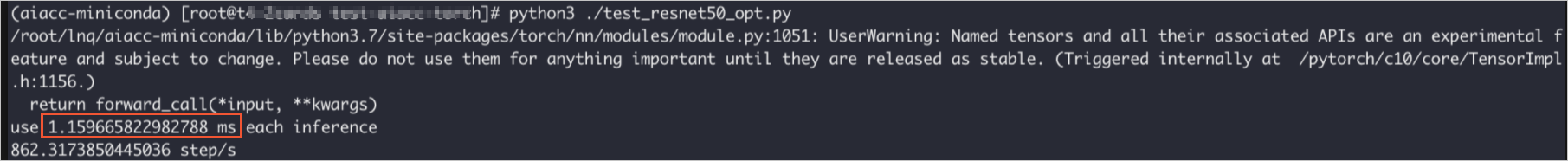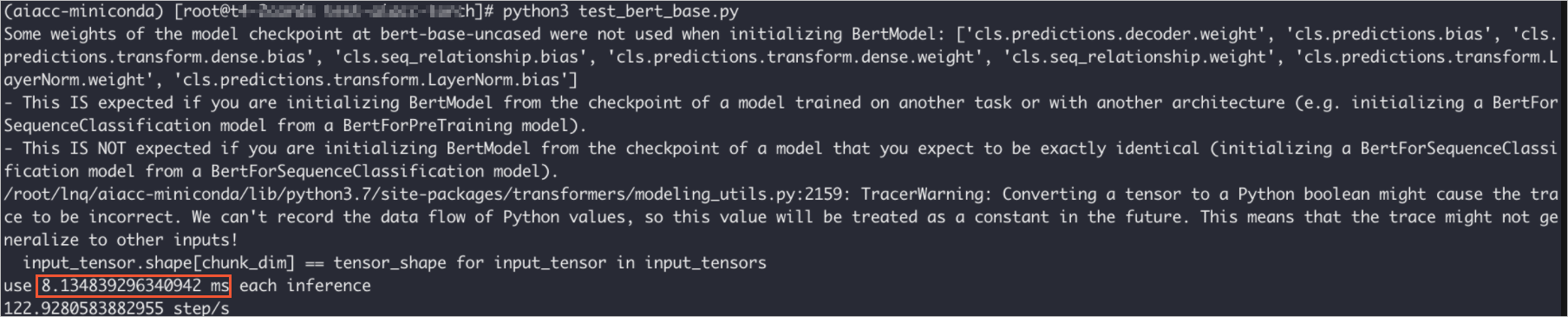AIACC-Inference can optimize models that are built based on the Torch framework to improve inference performance. This topic describes how to manually install AIACC-Inference in Torch and provides examples to demonstrate how AIACC-Inference accelerates inference operations.

Prerequisites

An Alibaba Cloud GPU-accelerated instance is created.
• Instance specifications: equipped with NVIDIA P100, V100, or T4 GPU
• The image used by the instance: Ubuntu 16.04 LTS or CentOS 7.x

Background information

AIACC-Inference in Torch cuts computational graphs of models, fuses layers, and makes high-performance operational to improve inference performance of PyTorch. You can optimize the inference performance of deep learning models in the PyTorch framework. When you optimize the inference performance, you can use just-in-time (JIT) compilation without specifying precision and dimensions.

AIACC-Inference in Torch can accelerate inferences by calling the aiacctorch.compile(model) operation. You need only to call the torch.jit.script or torch.jit.trace operation to convert the PyTorch model to the TorchScript model. For more information, see PyTorch official documentation. This topic provides examples on how to call the torch.jit.script and torch.jit.trace operations to improve inference performance.

Install AIACC-Inference in Torch

AIACC-Inference in Torch provides the Conda package, which can be installed with a few clicks, and the wheel package. You can select one of the packages for installation based on your business requirements.

• Conda package

The Conda package contains most of the dependency packages. You need only to manually install the CUDA driver and then install the Conda package. Perform the following operations:

Notice Do not change the information about the dependency packages that are pre-installed in the Conda package. If you change the information, an error may be reported due to version inconsistency when the demo runs.
1. Connect to a GPU-accelerated instance.
2. Install a CUDA driver of v455.23.05 or later.
wget https://aiacc-inference-torch.oss-cn-hangzhou.aliyuncs.com/aiacc-inference-torch-miniconda-latest.tar.bz2
4. Decompress the Conda package.
mkdir ./aiacc-inference-miniconda && tar -xvf ./aiacc-inference-torch-miniconda-latest.tar.bz2 -C ./aiacc-inference-miniconda
source ./aiacc-inference-miniconda/bin/activate
• Wheel package
You must manually install the dependency packages before you install the wheel package. Perform the following operations:
1. Connect to a GPU-accelerated instance.
2. Use one of the following methods to install the dependency packages. Select the dependency packages with caution because the wheel package depends on the combination of various software.
• Method 1
1. Install the following dependency packages:
• CUDA 11.1
• cuDNN 8.2.0.53
• TensorRT 7.2.3.4
2. Place the dependency libraries of TensorRT and CUDA in the LD_LIBRARY_PATH environment variable.

If the dependency libraries of CUDA are located in the /usr/local/cuda/ directory and the dependency libraries of TensorRT are located in the /usr/local/TensorRT/ directory, run the following commands. Replace the directories based on your business requirements.

export LD_LIBRARY_PATH=/usr/local/cuda/lib64:\$LD_LIBRARY_PATH
export LD_LIBRARY_PATH=/usr/local/TensorRT/lib:\$LD_LIBRARY_PATH
3. Execute the environment variable.
source ~/.bashrc
• Method 2

Use the NVIDIA pip to install dependency packages.

pip install nvidia-pyindex && \
pip install nvidia-tensorrt==7.2.3.4
3. Install PyTorch1.9.0+cu111.
• Python 3.6
wget https://aiacc-inference-torch.oss-cn-hangzhou.aliyuncs.com/aiacctorch-0.4.0a1-cp36-cp36m-linux_x86_64.whl
• Python 3.7
wget https://aiacc-inference-torch.oss-cn-hangzhou.aliyuncs.com/aiacctorch-0.4.0a1-cp37-cp37m-linux_x86_64.whl
• Python 3.8
wget https://aiacc-inference-torch.oss-cn-hangzhou.aliyuncs.com/aiacctorch-0.4.0a1-cp38-cp38-linux_x86_64.whl
• Python 3.9
wget https://aiacc-inference-torch.oss-cn-hangzhou.aliyuncs.com/aiacctorch-0.4.0a1-cp39-cp39-linux_x86_64.whl
5. Install the wheel package.
pip install *.whl

Perform inferences on the ResNet-50 model

In the following example, after the Conda package is installed, the torch.jit.script operation is called to perform inferences on the ResNet-50 model. The average time of 1,000 inferences is 1.15 ms, which is significantly reduced from the average inference time 9.12 ms before the AIACC-Inference in Torch (the Conda package) is installed.

• Original version

The following content shows the code before the acceleration.

import time
import torch
import torchvision.models as models
mod = models.resnet50()
mod_jit = torch.jit.script(mod)
mod_jit = mod_jit.cuda()

in_t = torch.randn([1,3,224,224]).float().cuda()

# Warming up
for _ in range(10):
mod_jit(in_t)

inference_count = 1000
# inference test
start = time.time()
for _ in range(inference_count):
mod_jit(in_t)
end = time.time()
print(f"use {(end-start)/inference_count*1000} ms each inference")
print(f"{inference_count/(end-start)} step/s")

The following result shows that the average inference time is about 9.12 ms.• Accelerated version

You need only to add the following lines to the sample code of the original version to accelerate inferences.

import aiacctorch
aiacctorch.compile(mod_jit)

The following content shows the code after the update:

import time
import aiacctorch #Import the AIACC-Inference in Torch package
import torch
import torchvision.models as models
mod = models.resnet50()
mod_jit = torch.jit.script(mod)
mod_jit = mod_jit.cuda()
mod_jit = aiacctorch.compile(mod_jit) #Make the compilation

in_t = torch.randn([1,3,224,224]).float().cuda()

# Warming up
for _ in range(10):
mod_jit(in_t)

inference_count = 1000
# inference test
start = time.time()
for _ in range(inference_count):
mod_jit(in_t)
end = time.time()
print(f"use {(end-start)/inference_count*1000} ms each inference")
print(f"{inference_count/(end-start)} step/s")

The following result shows that the average inference time is 1.15 ms. This result shows that the inference performance is significantly improved because the average inference time is reduced from the original 9.12 ms to 1.15 ms.Perform inferences on the Bert-Base model

In the following example, the torch.jit.trace operation is called to perform inferences on the Bert-Base model. The average inference time is reduced from 8.13 ms to 3.91 ms.

1. Install the transformers package.
pip install transformers
2. Run the demos of the original version and the accelerated version separately, and view the results.
• Original version

The following content shows the code before the acceleration.

from transformers import BertModel, BertTokenizer, BertConfig
import torch
import time

enc = BertTokenizer.from_pretrained("bert-base-uncased")

# Tokenizing input text
text = "[CLS] Who was Jim Henson ? [SEP] Jim Henson was a puppeteer [SEP]"
tokenized_text = enc.tokenize(text)

# Masking one of the input tokens
indexed_tokens = enc.convert_tokens_to_ids(tokenized_text)
segments_ids = [0, 0, 0, 0, 0, 0, 0, 1, 1, 1, 1, 1, 1, 1]

# Creating a dummy input
tokens_tensor = torch.tensor([indexed_tokens]).cuda()
segments_tensors = torch.tensor([segments_ids]).cuda()
dummy_input = [tokens_tensor, segments_tensors]

# Initializing the model with the torchscript flag
# Flag set to True even though it is not necessary as this model does not have an LM Head.
config = BertConfig(vocab_size_or_config_json_file=32000, hidden_size=768,

# Instantiating the model
model = BertModel(config)

# The model needs to be in evaluation mode
model.eval()

# If you are instantiating the model with `from_pretrained` you can also easily set the TorchScript flag
model = BertModel.from_pretrained("bert-base-uncased", torchscript=True)

model = model.eval().cuda()

# Creating the trace
traced_model = torch.jit.trace(model, dummy_input)

# Warming up
for _ in range(10):
all_encoder_layers, pooled_output = traced_model(*dummy_input)

inference_count = 1000
# inference test
start = time.time()
for _ in range(inference_count):
traced_model(*dummy_input)
end = time.time()
print(f"use {(end-start)/inference_count*1000} ms each inference")
print(f"{inference_count/(end-start)} step/s")

The following result shows that the average inference time is about 8.13 ms.• Accelerated version

You need only to add the following lines to the sample code of the original version to accelerate inferences.

import aiacctorch
aiacctorch.compile(traced_model)

The following content shows the code after the update:

from transformers import BertModel, BertTokenizer, BertConfig
import torch
import aiacctorch #Import the AIACC-Inference in Torch package
import time

enc = BertTokenizer.from_pretrained("bert-base-uncased")

# Tokenizing input text
text = "[CLS] Who was Jim Henson ? [SEP] Jim Henson was a puppeteer [SEP]"
tokenized_text = enc.tokenize(text)

# Masking one of the input tokens
indexed_tokens = enc.convert_tokens_to_ids(tokenized_text)
segments_ids = [0, 0, 0, 0, 0, 0, 0, 1, 1, 1, 1, 1, 1, 1]

# Creating a dummy input
tokens_tensor = torch.tensor([indexed_tokens]).cuda()
segments_tensors = torch.tensor([segments_ids]).cuda()
dummy_input = [tokens_tensor, segments_tensors]

# Initializing the model with the torchscript flag
# Flag set to True even though it is not necessary as this model does not have an LM Head.
config = BertConfig(vocab_size_or_config_json_file=32000, hidden_size=768,

# Instantiating the model
model = BertModel(config)

# The model needs to be in evaluation mode
model.eval()

# If you are instantiating the model with `from_pretrained` you can also easily set the TorchScript flag
model = BertModel.from_pretrained("bert-base-uncased", torchscript=True)

model = model.eval().cuda()

# Creating the trace
traced_model = torch.jit.trace(model, dummy_input)
traced_model = aiacctorch.compile(traced_model) #Make the compilation

# Warming up
for _ in range(10):
all_encoder_layers, pooled_output = traced_model(*dummy_input)

inference_count = 1000
# inference test
start = time.time()
for _ in range(inference_count):
traced_model(*dummy_input)
end = time.time()
print(f"use {(end-start)/inference_count*1000} ms each inference")
print(f"{inference_count/(end-start)} step/s")

The following result shows that the average inference time is 3.91 ms. This result shows that the inference performance is significantly improved because the average inference time is reduced from the original 8.13 ms to 3.91 ms.